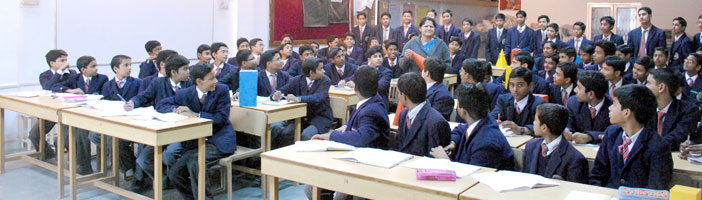# MATHEMATICS LABThe laboratory is named after great Mathematician “RAMANUJAM” for it embodies the spirit of exploration and teaches the child to observe keenly, reflect on ideas logically, think independently, innovatively and analyze intricately

Students from classes III to X learn Maths with models and practical projects in order to learn the subject in a simpler way. There are a numbers of experiments with colorful paper cutouts & paper folding activities which clarify concepts of lines, points, angles and triangles etc. of class VI as well as complex theorems of class X.

The computers and the internet are used for teaching, self learning, interactive mental Maths quizzes and speed calculation competitions. The geometric sketch pad and the handheld calculators are used to explain concepts from coordinate geometry to Calculus, to learn and understand quadratic equations; conic sections and trigonometric curves etc.